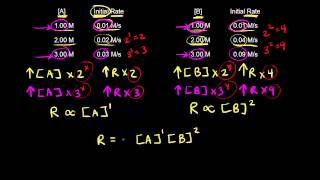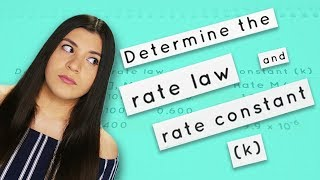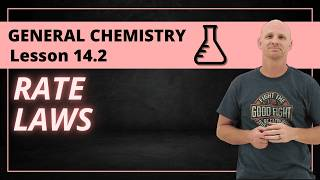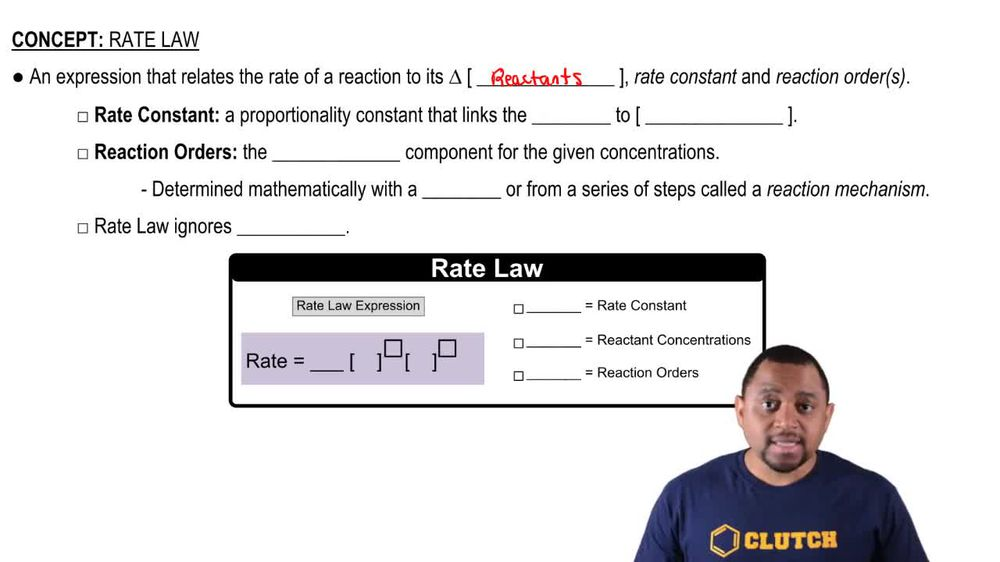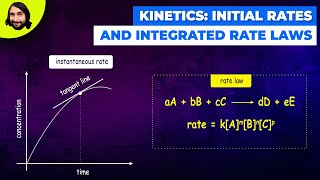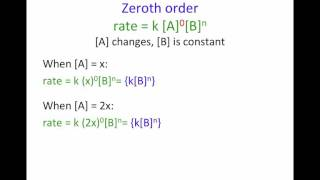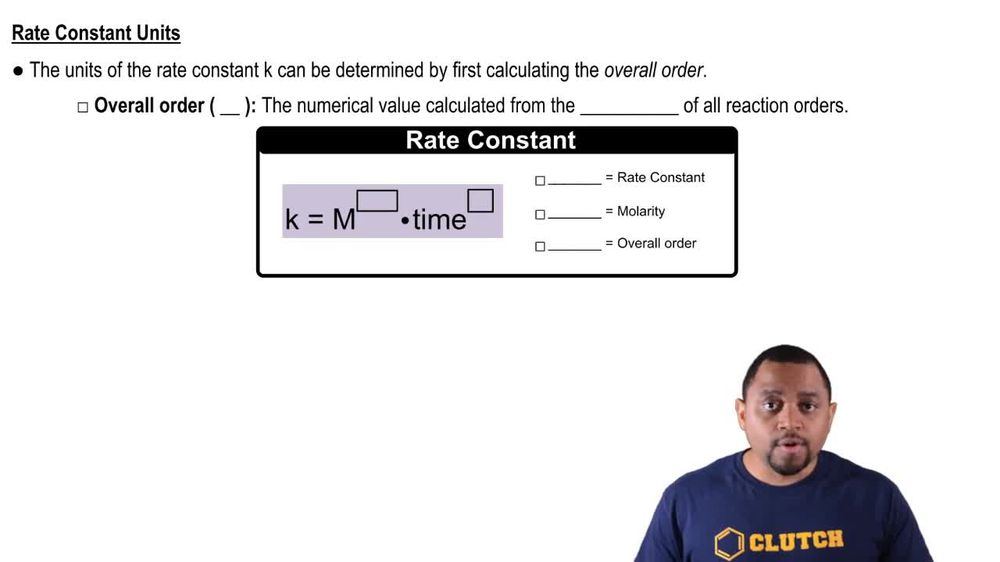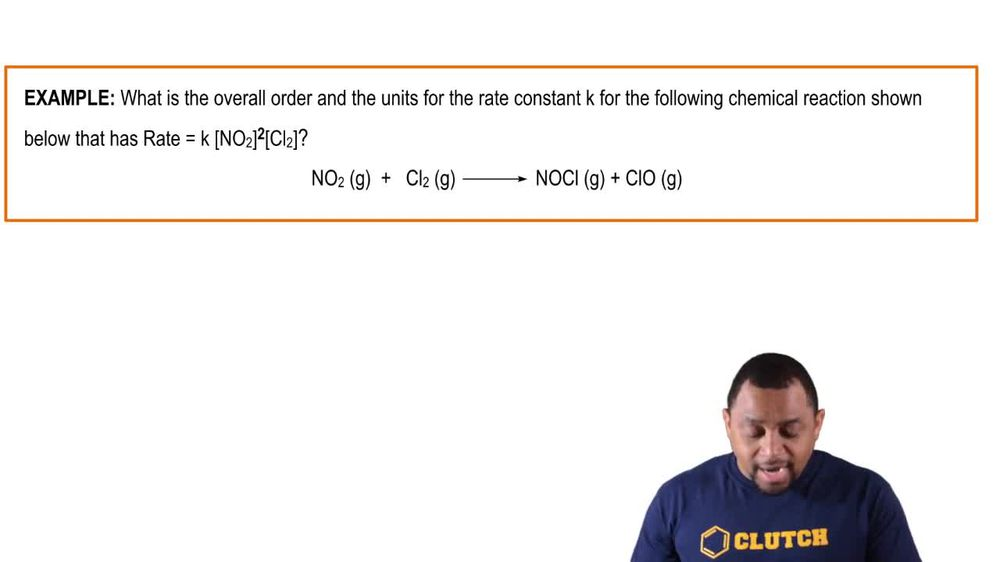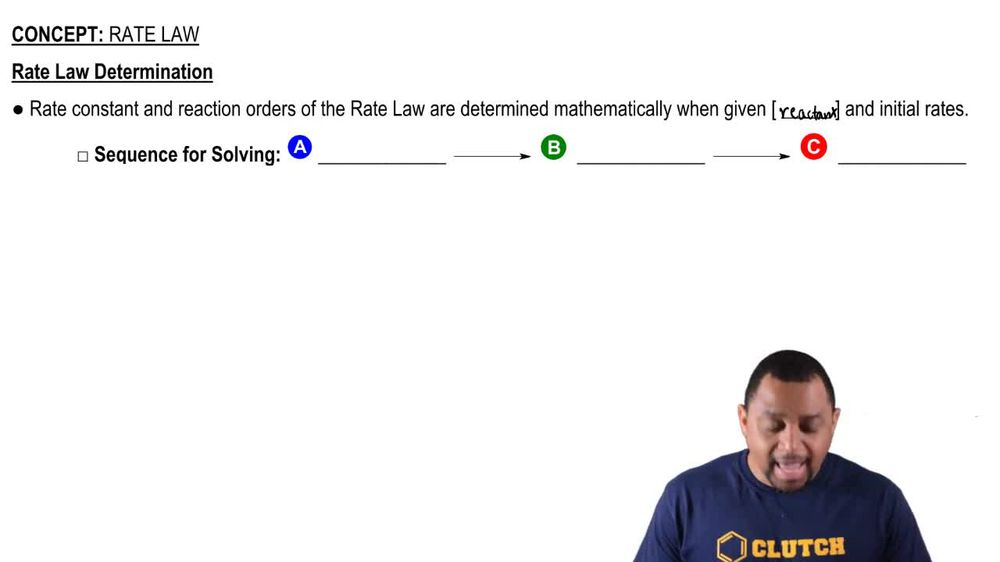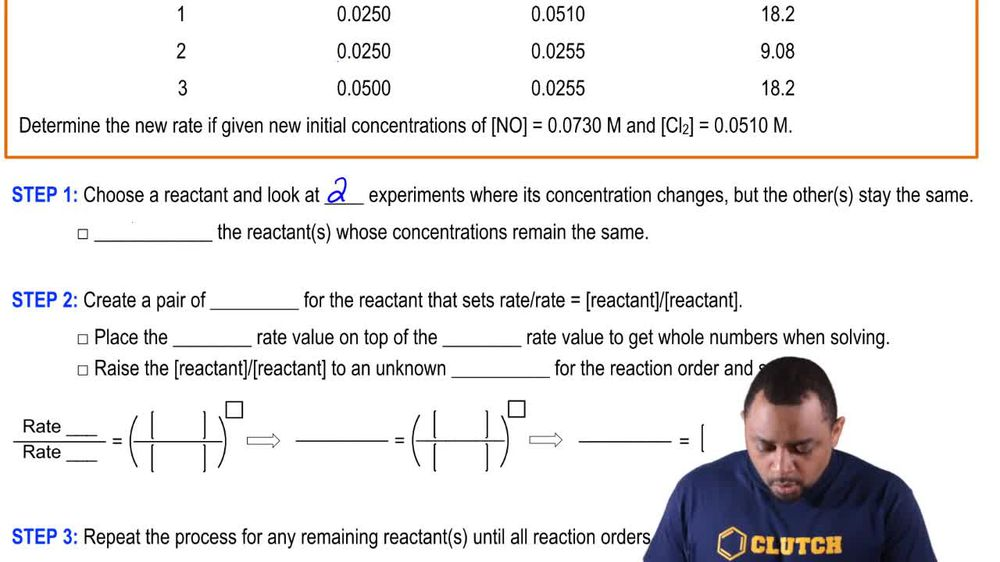Start typing, then use the up and down arrows to select an option from the list.
1. 15. Chemical Kinetics2. Rate Law# Rate Law Example 3

by Jules Bruno
183 views
2
0
the initial rates of reaction to N. O gas plus cl two gas giving us two N O C L gas are given below Here we have experiments +12 and three. And each experiment has attached to it reacting concentrations and initial rate. Now here it says determine the new rate if given new initial concentrations for NONCL two. Now, remember our sequence for solving is we first have to determine our reaction orders. Once we do that, then we can figure out our rate constant K. And then finally we can determine our new rate. Alright, so for step one, we're gonna choose a reactant and look at two experiments where its concentration changes, but the other reactions stay the same. These other reactant we're going to ignore. So you're gonna ignore the reactant whose concentrations remain the same here, we're gonna look for the reaction order of N O. So let's make it X. Cl two would have y you can make the many variables you want abc, whatever. Here, I just do X and Y. We have to look for two experiments where was changing but cl two is staying the same. That occurs with experiments two and 3 with experiments two and three. We cno is changing and the concentrations of cl two remain the same. Once we figured that out, we go to step two where we create a pair of ratios for the reactor that sets react rates equal to concentrations of the reactors. This is important. You place the larger rate value on top of the smaller rate value to get whole numbers when solving. And here you're going to raise the reacting concentrations to an unknown power or variable for the reaction order and solve. All right. So if we take a look here, experiments two and three we can see that rate three. Its rate initial rate three. Because it's connected to experiment three, it's larger than initial rate too. So that would mean that we're gonna have rape three over rape two equals the concentrations of N. O. To the X. Now we're gonna plug in the number. So 18.2 divided by 9.08 equals 0.500 divided by 0.0 to 50. Still to the X 18.2 divided by 9.08 is approximately two And then .0500 divided by .0250 is two and it's still 50 x. Now you say to to what number gives you to the answer would be one. So the reaction order for N. O. Is one. Now we're gonna do the same thing for step three. We're going to repeat the process for any remaining reactant until all reaction orders are determined. Right? So now we have to look for cl two. We look for two experiments where cl two is changing but N. O. Is staying the same. We see that happen with experiments one and 2. Now here initial rate one is larger than initial rate too. So if we come down here we're gonna say rate one over rate two equals C. L. Two divided by C. L. Two to the Y. So let's see that was 18.2 18.2 divided by 9.8. And then here we're gonna plug in the concentrations for cl two. So here for cl two it's 20.510 and this is 0.255 to the wat. This also comes out to two equals 2 to the Y. To to what number gives us to. The answer is one. So it's first order for both of our react it's now step forward if necessary. Here it is necessary because we need to find our new rate to find our new rate we have to figure out our react our rate constant. So here we're gonna say if necessary to solve for the rate constant. K. Plug in the reacting concentration and reacting orders into the rate law. Alright so here we're going to say that our rate We're gonna say rate one. You can use any of the rates but just to keep it simple. Always go with rate one. So rate one equals Kay And we're gonna stay here n. O to the one Cl 2 to the one. Okay and then we're gonna start plugging the numbers. So 18.2 we're looking for Kay we don't know what K. Is. Come back up here. Let's use the concentration for. So since we're using rate one we have to use the concentrations of experiment one. So that's 10.0 to 50 and 500.510 To the one in .0510 to the one. Alright so here when we multiply these together that's gonna give me point .001275. Still multiplying with K. rate one again is 18.2 divide both sides by 0.1275. And we'll have our K. Here K equals 14117.647. Now here we could technically give the units for cake because remember to find the units of K. It's M to the negative end plus one times time in verse. And here's our overall order which is two and it comes from adding up your reaction orders together. So that's negative two plus one. Here let's just use seconds in verse. Since our initial rates are polarities per second. So that would be M to the negative one times seconds inverse. Okay finally we can figure out our new rate and again if necessary we're gonna say to solve for the new rate plug in the k. The reaction orders and the additional reaction concentrations given. So here we're going to say that our new rate equals K. And we're gonna plug in the new concentrations given to us in the very beginning. So let's come up here. So here we have 0.730 for R N O. And then we have 00.510 for our cl two. So those are the new concentrations we're gonna plug into here, So let's come down here. So this is .0730 actually. And that's again for N. O. To the one in C. 02 to the one. So new rate. So okay, we got is 14117.647 N O. Is 00.730 to the one cl two is 20.510 to the one when we plug all that in. That gives us 52.6 polarities per second for a new rate. So we can see that if they're asking us for the new rate, it is quite an ordeal. You have to go through a lot of things to get to your final answer. Remember the sequence of solving is important. First figure out reaction orders before you can figure out your rate constant K. Before you can figure out your final new rate